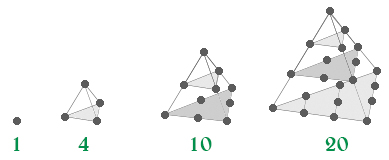# Tetrahedral Numbers

• Last Updated : 05 Apr, 2021

A number is termed as a tetrahedral number if it can be represented as a pyramid with a triangular base and three sides, called a tetrahedron. The nth tetrahedral number is the sum of the first n triangular numbers.
The first ten tetrahedral numbers are:
1, 4, 10, 20, 35, 56, 84, 120, 165, 220, …Attention reader! Don’t stop learning now. Get hold of all the important mathematical concepts for competitive programming with the Essential Maths for CP Course at a student-friendly price. To complete your preparation from learning a language to DS Algo and many more,  please refer Complete Interview Preparation Course.

Formula for nth tetrahedral number:

`Tn = (n * (n + 1) * (n + 2)) / 6`

Proof:

```The proof uses the fact that the nth tetrahedral
number is given by,

Trin = (n * (n + 1)) / 2

It proceeds by induction.

Base Case
T1 = 1 = 1 * 2 * 3 / 6

Inductive Step
Tn+1 = Tn + Trin+1

Tn+1 = [((n * (n + 1) * (n + 2)) / 6] + [((n + 1) * (n + 2)) / 2]

Tn+1 = (n * (n + 1) * (n + 2)) / 6```

Below is the implementation of above idea :

## C++

 `// CPP Program to find the``// nth tetrahedral number``#include ``using` `namespace` `std;` `int` `tetrahedralNumber(``int` `n)``{``    ``return` `(n * (n + 1) * (n + 2)) / 6;``}` `// Driver Code``int` `main()``{``    ``int` `n = 5;``    ` `    ``cout << tetrahedralNumber(n) << endl;` `    ``return` `0;``}`

## Java

 `// Java Program to find the``// nth tetrahedral number``class` `GFG {``    ` `// Function to find Tetrahedral Number``static` `int` `tetrahedralNumber(``int` `n)``{``    ``return` `(n * (n + ``1``) * (n + ``2``)) / ``6``;``}` `// Driver Code``public` `static` `void` `main(String[] args)``{``    ``int` `n = ``5``;``    ` `    ``System.out.println(tetrahedralNumber(n));``}``}` `// This code is contributed by Manish Kumar Rai.`

## Python

 `# Python3 Program to find the``# nth tetrahedral number` `def` `tetrahedralNumber(n):``    ` `    ``return` `(n ``*` `(n ``+` `1``) ``*` `(n ``+` `2``)) ``/` `6` `# Driver Code``n ``=` `5``print` `(tetrahedralNumber(n))`

## C#

 `// C# Program to find the``// nth tetrahedral number``using` `System;` `public` `class` `GFG{``    ` `    ``// Function to find Tetrahedral Number``    ``static` `int` `tetrahedralNumber(``int` `n)``    ``{``        ``return` `(n * (n + 1) * (n + 2)) / 6;``    ``}``    ` `    ``// Driver code``    ``static` `public` `void` `Main ()``    ``{``        ``int` `n = 5;``    ` `        ``Console.WriteLine(tetrahedralNumber(n));``    ``}``}` `// This code is contributed by Ajit.`

## PHP

 ``

## Javascript

 ``

Output:

`35`

Time Complexity: O(1).

My Personal Notes arrow_drop_up